# Similarity Distance Measures

## Similarity Measures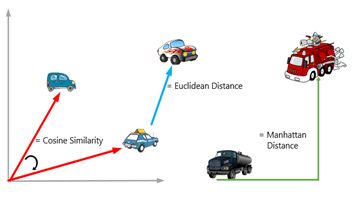Similarity or Similarity distance measure is a basic building block of data mining and greatly used in Recommendation Engine, Clustering Techniques and Detecting Anomalies.

By definition, Similarity Measure is a distance with dimensions representing features of the objects. In a common term, this is a measure which helps us identify how much alike two data objects are. If the distance is small, the objects have high similarity factor and vice versa. So if two objects are similar they are denoted as Obj1 = Obj2 and if they are not similar they are denoted as Obj1 != Obj2

The similarity is always measured in the range of 0 to 1 and is denoted as [0,1].

There are various techniques of calculating Similarity Distance measure. Let’s look at some of the most popular one.

## Euclidean distance

This is most commonly used measure and is denoted as

Similarity Distance Measure = SQRT ((xB-xA)^2+ (yB-yA)^2) )

The Euclidean distance between two points is the length of the path connecting them.

Let’s take a simple example –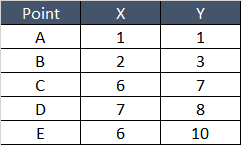Let’s Plot these points on Graph and see how do they look –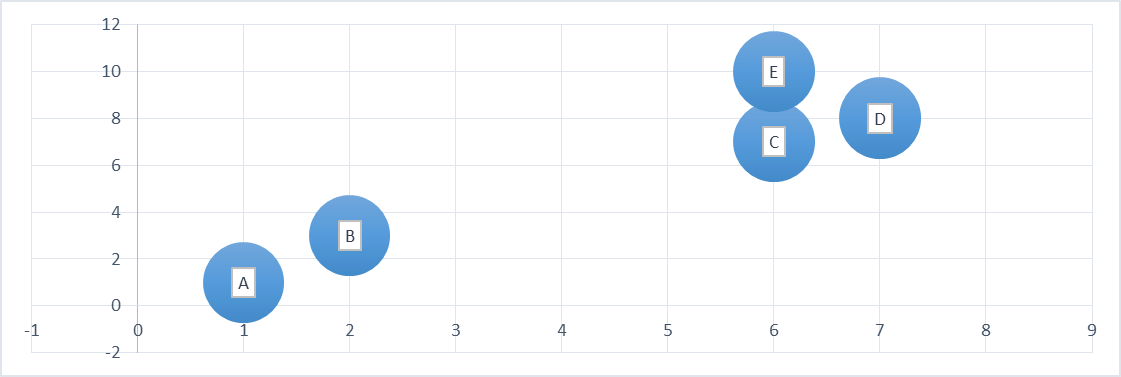Below table shows Distance between any two points –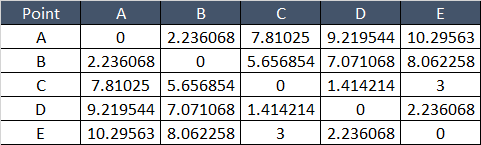As the Similarity measure is always between 0 and 1, let’s convert the distance into measure with the formula –

d_normalized = d / max (distance) and then

Similarity = 1- d_normalized

Below table gives Similarity score for any two points –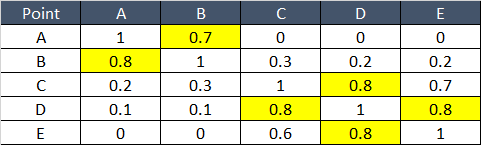From above table B is nearest to A, C and D are near & E and D are near.

## Manhattan distance

This is one more commonly used measure and is denoted as:

Similarity Distance Measure = Abs (xB-xA) + Abs(yB-yA)

If we continue to consider above points A, B, C, D and E, then Manhattan Distance calculation would be something like below –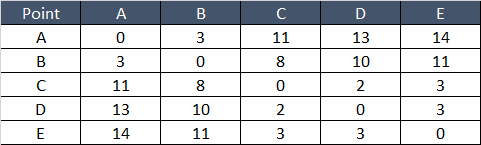And then following above steps Normalising Distance and Similarity Score.

## Minkowski Distance

This is one more method of Calculating Distance and its mix of above two. Just value of p = 1 then it becomes Manhattan and p = 2 then it becomes Euclidean.

Minkowski Distance = (Xi – Yi)^p

## Jaccard Similarity

When we need to identify similarity between two sets, Jaccard Similarity Metric is used.

This is denoted as – Intersection of two sets / Union of two sets

Set is nothing but collection of objects.

Carnality of a set is denoted as count of elements contained in that set. Intersection between two sets is no. of common items in both the sets. Union is all items which are present in either of set

E.g. we need to find out how similar below sets are –

Set A = [Bread, Milk, Butter, Eggs, Cheese, Beer]

Set B = [Milk, Bread, Egg, Onion, Tomato, Butter, Cheese, Beer]

Set C = [Shirt, Mobile, Juice, Beer, Egg, Cream, Chocolate]

Union [A,B] = [Bread, Milk, Butter, Eggs, Cheese, Beer, Onion, Tomato]

Intersection of [A,B] = [Bread, Milk, Butter, Eggs, Cheese, Beer]

JS [A,B] = Intersection of [A,B] / Union [A,B]

JS [A,B] = 6 / 8

JS [A,B] = 0.75

Let’s check similarity of Sets A and C –

Union [A,C] = [Bread, Milk, Butter, Eggs, Cheese, Beer, Shirt, Mobile, Juice, Cream, Chocolate]

Intersection of [A,C] = [Eggs, Beer]

JS [A,B] = Intersection of [A,B] / Union [A,B]

JS [A,B] = 2 / 11

JS [A,C] = 0.18

Sets [A,B] are 0.75 similar whereas Set [A,C] are 0.18 similar

## Cosine Similarity

Using this technique, we find the Cosine of angle between the two points (Draw line from Origin to Point A and then Point B and See what is the angle between these two lines are Origin). So Cosine of 0 is 1 and Cosine of 90 is 0.

This can also be termed as when two points are same the angle would be 0 and so they can be termed as similar points since their angle is 0 and Cosine of 0 is 1. If Points are diametrically opposite – it would be Cosine of 180 which is -1.

Cosine Similarity is used mainly in positive space.

Similarity score is calculated for each point against every other point and then it’s decided which two points are close and can go together. Some of the commercial examples for recommendation system is – “What product can be recommended to user based on user’s preferences”.

Please do visit our blog on Recommendation Engine for details.